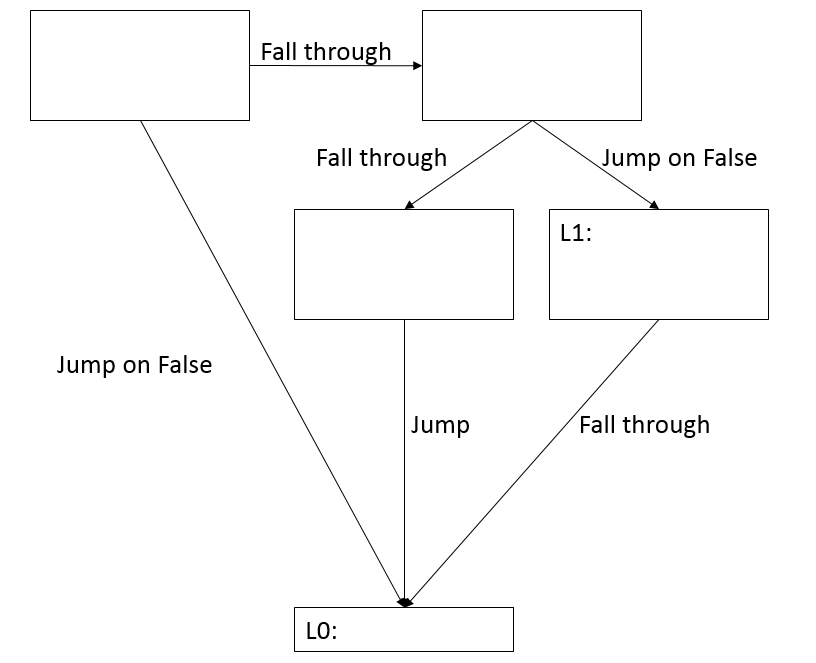## Homework 5

### Question 1:

Assume we have a language that allows arrays and that parameters in this language can be passed by value, by reference, by value-result, or by name. Consider the following program:
```    A;
int k;

void f( int x, int y ) {
k = k * 2;
y = y + 2;
A[k] = A[k] - 1;
x = A[k] - 2;
cout << x;
cout << y;
cout << k;
cout << A[k];
}

void main() {
k = 0;
while (k < 4) {
A[k] = k * 2;
k = k + 1;
}
k = 1;
f(k, A[k]);
cout << k;
cout << A;
cout << A;
cout << A;
cout << A;
}
```

Below is a link to a file that contain six pictures. The first four pictures contain outlines of f's activation record, as well as the space in the static data area for globals k and A. The last two pictures contain space for recording the output of the program.

• For the first four pictures, you should fill in the values for all of the variables as they would be just before function f returns. For each picture, assume the parameter-passing modes for f's parameters indicated in that picture.

• For the last two pictures, you should fill in the values that would be printed by functions f and main, assuming that f's parameters are both passed by value-result or both passed by name (as indicated in the pictures).

### Question 2:

In class, we talked about how a control-flow graph represents control transfer in terms of edges on a graph. In this question, you will first generate the MIPS code, then fill your MIPS code into the control flow graph below. You can assume a, b and c are global variables, You can access value of variable a as: "lw \$t0, a". List any other assumptions you are making. Please only use register \$t0 and \$t1.

Block of code:
```if (a < c) {
if (b == c) {
b = a;
}
else {
c = a;
}
}
```### Question 3:

For the final project, you generate code for expressions as discussed in class: the codeGen method for each kind of expression will generate code to evaluate the expression, leaving the value on the stack.

While this kind of code is easy to generate, storing intermediate values on the stack rather than in registers is inefficient. You might think we could instead require that the codeGen method for an expression should work as follows:

1. Each expression node's codeGen method would have 1 parameter: a register number N, which would be either 0 or 1.
2. For literals or identifiers, the codeGen method would simply load the appropriate value in register N.
3. For expressions involving non short-circuited binary operators (+, *, <, etc.) the codeGen method would call the codeGen method of the left child with argument 0 (which would generate code to evaluate the left expression, leaving the result in register 0), then call the codeGen method of the right child with argument 1 (which would generate code to evaluate the right expression, leaving the result in register 1), then perform the operation, leaving the result in the appropriate register.

Unfortunately, this approach does not always work.

Assume that the AST includes only expressions that involve non short-circuited binary operators, with literals or identifiers at the leaves (no unary operators, no dot-access expressions or function calls as operands). Also assume that in the generated code, all operands must be in registers (i.e., neither operand of a binary operator can be in a memory location, nor can it be a literal value).

For the following examples, judge whether the approach described above works. If not, show at least how many registers we need to evaluate the expression, the AST of expression and a pseudo-code version of the code to evaluate the expression (code that works, not the erroneous code that would be generated using the approach described above). If the approach described above works, you can just answer "Yes" and do not need to show the AST and pseudo-code. You can assume that no optimization is done prior to code generation.

1. (a-b) - c

2. (a-b) - (c-d)

3. ((a - b) - (c - d)) - ((e - f) - (g - h))

Here is a example of AST and pseudo-code for the expression a-b (use the same kind of pseudo-code in your answer):

 Expression AST pseudo code `a - b` ``` - / \ a b ``` ``` load a into T0 load b into T1 T0 = T0 - T1 ```

Note that an expression's operands need not be evaluated left-to-right. For example, the following pseudo-code would also be OK for the expression a - b:

```    load b into T1    // evaluate the right operand first